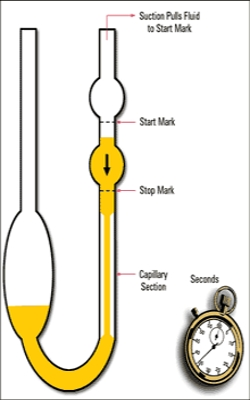Chemistry

# Measurement of Viscosity

Measurement of Viscosity

There are a number of diverse methods for determining the co-efficient of viscosity: for example, (a) by determining the volume of liquid flowing through a capillary of known dimensions under a given pressure, i.e., using Poiseuille’s equation; (b) from the rate of fall of spherical balls through the liquid; (c) from the torsion in a standard wire supporting a cylinder immersed in a liquid moving at a known speed.

The method most useful for ordinary liquids is that based on Poiseuille’s equation.

It is difficult to determine the absolute viscosity by Poiseuille’s method. The usual procedure is to determine the viscosity of given liquid relative to another liquid whose viscosity, is accurately known. Such relative measurement is a common practice in the determination of many physic-chemical quantities.Fig: Simple Viscometer

A relatively simple viscometer as shown in Figure, may be used for the determination of relative viscosities. For a given viscometer the radius, R, and length, l, are constants. The pressure difference that causes the liquid to flow through the capillary is the difference, h, between the levels of liquids in the bulbs. If d is the density of the liquid this pressure will be h, g and d.

For a liquid of density, d1, and viscosity, η1, if the time of flow through the capillary is t1 seconds, equation (Poiseuille’s) takes the form;

η1 = [(hg π R4) / (8Vl)] d1t1

If the same volume of another liquid of viscosity, η2, and density. d2, flows through the capillary in the same viscometer in t2 seconds, then

η2 = [(hg π R4) / (8Vl)] d2t2

The ratio of viscosities is then given by:

η1/ η2 = d1t1/d2t2 … …. (1)

Water is usually taken as the standard substance. It is obvious that if the viscosity of one liquid is known, that of the ether may be calculated from the measured values of the densities and times of flow of the two liquids.

The viscometer shown in Figure, as known as the Ostwald viscometer. A known appropriate volume of the liquid under examination is first placed in the wider limb and sucked up the capillary to a level beyond the upper mart in the capillary. The liquid is then allowed to fall and the time taken for the liquid level to tall from the upper to the lower mark in the capillary is noted. The liquid is then replaced by an equal volume of the second liquid and the procedure is repealed. Use of equation (1) will give the required viscosity value. There are a number of other viscometers in use which are simple modifications of the Ostwald viscometer.